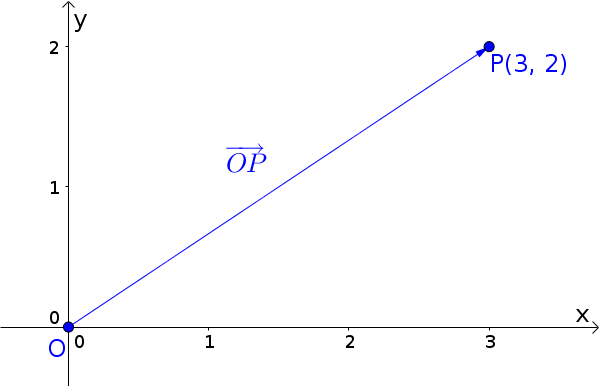Math Vectors Position vector

# Position vector

For the position vector $\overrightarrow{OP}$ of a point $P(p_1|p_2)$ , the initial point always lies in the coordinate origin $O(0|0)$.
The coordinates of the vector therefore correspond to those of the point.

$\overrightarrow{OP}=\begin{pmatrix}p_1\\p_2\end{pmatrix}$

In three-dimensional space with $P(p_1|p_2|p_3)$:

$\overrightarrow{OP}=\begin{pmatrix}p_1\\p_2\\p_3\end{pmatrix}$
i

### Hint

The position vector is a vector between two points with the origin as the initial point.

### Example

Position vector $\overrightarrow{OP}$ of $P(3|2)$$\overrightarrow{OP}=\begin{pmatrix}3\\2\end{pmatrix}$